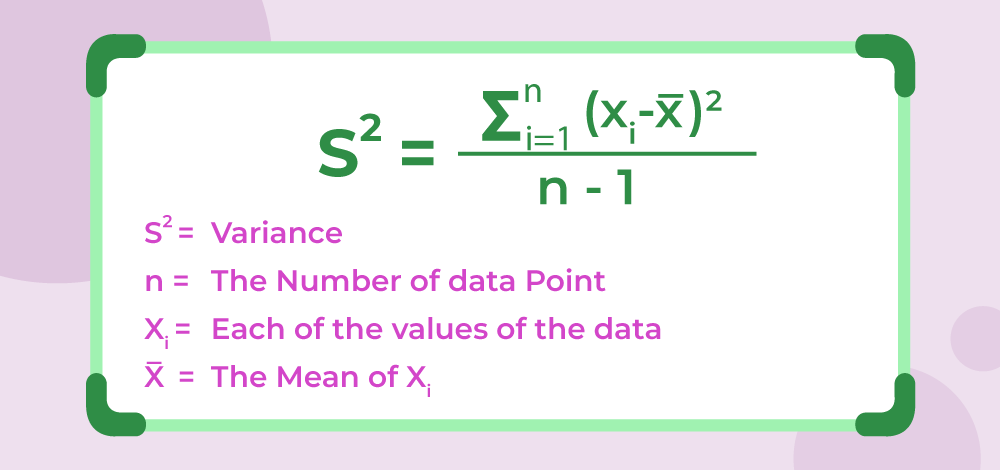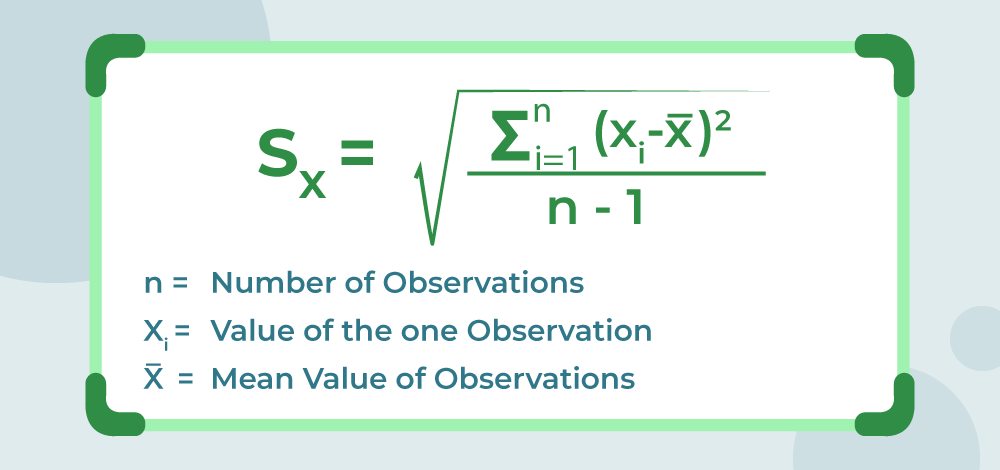Related Articles

# Variance and Standard Deviation

Variance and Standard Deviation are the important measures used in Mathematics and Statics to find the meaning from a large set of data. The different formulas for Variance and Standard Deviation are highly used in mathematics to determine the trends of various values in mathematics. Variance is the measure of how the data points vary according to the mean while standard deviation is the measure of the central tendency of the distribution of the data.

The major difference between variance and standard deviation is in their units of measurement. Standard deviation is measured in a unit similar to the units of the mean of data, whereas the variance is measured in squared units. Here in this article, we will learn about variance and standard deviation including their definitions, formulas, and their differences along with suitable examples in detail.

## Variance

Variance is defined as, “The measure of how far the set of data is dispersed from their mean value”. Variance is represented with the symbol σ2. In other words, we can also say that the variance is the average of the squared difference from the mean.

### Properties of Variance

Various properties of the Variance of the group of data are,

• As each term in the variance formula is firstly squared and then their mean is found, it is always a non-negative value, i.e. mean can be either positive or can be zero but it can never be negative.
• Variance is always measured in squared units. For example, if we have to find the variance of the height of the student in a class, and if the height of the student is given in cm then the variance is calculated in cm2

## Variance Formula

There are two formulas for Variance, that are:

• Population Variance
• Sample Variance

### Formula for Population Variance

The mathematical formula to find the variance of the given data is,Where,

• σ2 is the Variance of the Population,
• N is the Number of Observation in the Population,
• Xi is the ith observation in the Population, and
• μ is the mean of the Population.

This formula is also called the Population variance formula as it is used for finding the variation in the population data.

### Formula for Sample Variance

Also, the other formula for finding the variance is the sample variance formula is discussed in the image## Standard Deviation

How far our given set of data varies along with the mean of the data is measured in standard deviation. Thus, we define standard deviation as the “spread of the statistical data from the mean or average position”. We denote the standard deviation of the data using the symbol σ.

We can also define the standard deviation as the square root of the variance.

### Properties of Standard Deviation

Various properties of the Variance of the group of data are,

• Standard Deviation is the square root of the variance of the given data set. It is also called root mean square deviation.
• Standard Deviation is a non-negative quantity i.e. it always has positive values or zero values.
• If all the values in a data set are similar then Standard Deviation has a value close to zero. Whereas if the values in a data set are very different from each other then standard deviation has a high positive value.

## Standard Deviation Formula

There are two formulas for the standard deviation listed as follows:

• Population Standard Deviation
• Sample Standard Deviation

### Formula for Population Standard Deviation

The mathematical formula to find the standard deviation of the given data is,

s =Where,

• σ is the Standard Deviation of the Population,
• N is the Number of Observation in the Population,
• Xi is the ith observation in the Population, and
• μ is the mean of the Population

This formula is also called the Population standard deviation formula as it is used for finding the standard deviation in the population data.

### Formula for Sample Standard Deviation

Also, the other formula for finding the standard deviation is the sample space i.e. sample variance formula is discussed in the image below,## Relation between Standard Deviation and Variance

Variance and Standard deviation are the most common measure of the given set of data. They are used to find the deviation of the values from their mean value or the spread of all the values of the data set.

• Variance is defined as the average degree through which all the values of a given data set deviate from the mean value.
• Standard Deviation is the degree to which the values in a data set are spread out with respect to the mean value.

The relationship between Variance and Standard Deviation is discussed below.

Variance = (Standard Deviation)2

OR

√(Variance) = Standard Deviation

## Differences Between Standard Deviation and Variance

The differences between Standard Deviation and Variance are discussed in the table below,

## Examples on Variance and Standard Deviation

Example 1: Find the variance and standard deviation of all the possibilities of rolling a die.

Solution:

All possible outcomes of rolling a die are {1; 2; 3; 4; 5; 6}.

This data set has six values (n) = 6

Before finding the variance, we need to find the mean of the data set.

Mean, x̅ = (1+2+3+4+5+6)/6 = 3.5

We can put the value of data and mean in the formula to get;

σ2 = Σ (xi – x̅)2/n

⇒ σ2 = [(1-3.5)2 + (2-3.5)2 + (3-3.5)2 + (4-3.5)2 + (5-3.5)2 + (6-3.5)2]/6

⇒ σ2 = (6.25+2.25+0.25+0.25+2.25+6.25)/6

Variance (σ2) = 2.917

Now,

Standard Deviation (σ) = √ (σ2)

⇒ Standard Deviation (σ)  = √(2.917)

⇒ Standard Deviation (σ) = 1.708

Example 2: Find the variance and standard deviation of all the even numbers less than 10.

Solution:

Even Numbers less than 10 are {0, 2, 4, 6, 8}

This data set has five values (n) = 5

Before finding the variance, we need to find the mean of the data set.

Mean, x̅ = (0+2+4+6+8)/5 = 4

We can put the value of data and mean in the formula to get;

σ2 = Σ (xi – x̅)2/n

⇒ σ2 = [(0-4)2 + (2-4)2 + (4-4)2 + (6-4)2 + (8-4)2]/5

⇒ σ2 = (16 + 4 + 0 + 4 + 16)/5 = 40/5

Variance (σ2) = 8

Now, Standard Deviation (σ) = √ (σ2)

⇒ Standard Deviation (σ)  = √(8)

⇒ Standard Deviation (σ) = 2.828

## FAQs on Variance and Standard Deviation

### Q1: What Does Variance Mean?

Variance is defined as the statistical measurement which determines how far each number is from the mean value in a given data set. It tells us about the degree to which our average value can deviate in the data set.

### Q2: What Does Standard Deviation Mean?

Standard deviation is the measure of the volatility from the mean value in the given data set. It is calculated by taking the square root of the variance of the given data set. It calculates the risk related to the change in values in the market.

### Q3: What Is the Difference Between Standard Deviation and Variance?

Variance is calculated by taking the average of the squared deviation from the mean, whereas standard deviation is the square root of the variance. The other difference between them is in their unit. Standard deviation is expressed in the same units as the original values while Variance is expressed in unit2.

### Q4: What is Variance formula?

The formula to calculate the variance of the given data set is,

σ2 = Σ (xi – x̅)2/n

Where,

• σ2 is the Variance of the data,
• is the mean of the data, and
• n is the number of values of the data.

### Q5: What is Standard Deviation formula?

The formula to calculate the variance of the given data set is,

σ = √(Σ (xi – x̅)2/n)

Where,

• σ is the Variance of the data,
• is the mean of the data, and
• n is the number of values of the data.